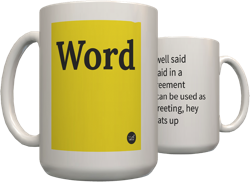2
There are at least two usages of "trivial". One is to describe answers, and the other is to describe questions/tasks.

Answers: A trivial answer is something which is either immediately self-evident or which conveys no information.

Questions/Tasks: A trivial question can perhaps be defined as something for which either the answer or the method of finding the answer is known by the person asked the question.
Answers: When asked to describe yourself, a nontrivial answer would be, "My name is Joe Schmoe. I'm 5'10" and weigh 170 lbs." A trivial answer would be, "I'm a human. I know English."

Questions/Tasks: In mathematics, computing the values of integrals is a trivial task, (even though it can be hard), because the methods for computing integrals are known.
by Alexander TG April 29, 2006Get a trivial mug for your Uncle Bob.